# 零膨胀几何分布的变量选择Variable Selection of Zero-Inflated Geometric Distribution

DOI: 10.12677/AAM.2021.104135, PDF, HTML, XML, 下载: 7  浏览: 25

Abstract: In health services and outcome research, count results are often encountered, and there is usually a large proportion of zeros. The zero-inflated geometric regression model is a powerful tool for analyzing excessive zeros in geometrical parts. In actual modeling, there may be variables that are completely unrelated to the target (redundant variables) among the collected variables, or some variables are known to be related to the target, but the actual impact is minimal. Aiming at the problem of many covariates and correlations, this paper adds SCAD, MCP and LASSO penalties to the likelihood function to obtain a penalty objective function based on zero-inflated geometric regression, and then uses the EM algorithm to study the parameter estimation and variable selection of the model problem. Simulation research shows that the model not only has accurate parameter estimation, but also is superior to the traditional stepwise selection method.

1. 引言

2. 惩罚ZIGe回归模型

2.1. 惩罚ZIGe回归模型的变量选择

$P\left({Y}_{i}={y}_{i}\right)=\left\{\begin{array}{l}{p}_{i}+\left(1-{p}_{i}\right)\left(1-{\theta }_{i}\right),\text{}{y}_{i}=0\hfill \\ \left(1-{p}_{i}\right)\left(1-{\theta }_{i}\right){\theta }_{i}{}^{{y}_{i}},\text{}{y}_{i}=1,2,\cdots \hfill \end{array}$ (1)

$\left\{\begin{array}{c}logit\left({p}_{i}\right)={W}_{i}^{\text{T}}\gamma \\ logit\left({\theta }_{i}\right)={X}_{i}^{\text{T}}\beta \end{array}$ (2)

$\begin{array}{c}\mathcal{l}\left(\phi ;{y}_{i},{x}_{i},{w}_{i}\right)=\underset{i=1}{\overset{n}{\sum }}\mathrm{log}\left\{{I}_{\left({y}_{i}=0\right)}\left[\frac{{p}_{i}}{\left(1-{p}_{i}\right)}+\left(1-{\theta }_{i}\right)\right]\left(1-{p}_{i}\right)+{I}_{\left({y}_{i}>0\right)}\left[{\theta }_{i}{}^{{y}_{i}}\left(1-{\theta }_{i}\right)\right]\left(1-{p}_{i}\right)\right\}\\ =\underset{i=1}{\overset{n}{\sum }}\mathrm{log}\left(\mathrm{exp}\left({W}_{i}{}^{\text{T}}\gamma \right)+{\left(1+\mathrm{exp}\left({X}_{i}{}^{\text{T}}\beta \right)\right)}^{-1}\right)-\mathrm{log}\left(1+\mathrm{exp}\left({W}_{i}{}^{\text{T}}\gamma \right)\right)\\ +\underset{i=1}{\overset{n}{\sum }}{I}_{\left({y}_{i}>0\right)}\left[{y}_{i}{X}_{i}{}^{\text{T}}\beta -\left({y}_{i}+1\right){\left(1+\mathrm{exp}\left({X}_{i}{}^{\text{T}}\beta \right)\right)}^{-1}\right]\end{array}$ (3)

$p\mathcal{l}\left(\phi ;{y}_{i},{x}_{i},{w}_{i}\right)=\mathcal{l}\left(\phi ;{y}_{i},{x}_{i},{w}_{i}\right)-p\left(\beta ,\gamma \right)$ (4)

$p\left(\beta ,\gamma \right)=n{\sum }_{j=1}^{{p}_{1}}{p}_{{a}_{j}}|{\beta }_{j}|-n{\sum }_{k=1}^{{p}_{2}}{p}_{{b}_{k}}|{\gamma }_{k}|$ (5)

${p}_{\lambda }\left(\xi \right)=\left\{\begin{array}{l}\lambda |\xi |,\text{}|\xi |\le \lambda \hfill \\ \frac{\left({a}^{2}-1\right){\lambda }^{2}-{\left(|\xi |-a\lambda \right)}^{2}}{2\left(a-1\right)},\text{}\lambda <|\xi |\le a\lambda \hfill \\ \frac{\left(a+1\right){\lambda }^{2}}{2},\text{}|\xi |>a\lambda \hfill \end{array}$ (6)

${{p}^{\prime }}_{\lambda }\left(\xi \right)=\left\{\begin{array}{l}\lambda ,\text{}|\xi |\le \lambda \hfill \\ \frac{a\lambda -\xi }{a-1},\text{}\lambda <|\xi |\le a\lambda \hfill \\ 0,\text{}|\xi |>a\lambda \hfill \end{array}=\lambda \left\{I\left(\xi \le \lambda \right)+\frac{{\left(a\lambda -\xi \right)}_{+}}{\left(a-1\right)\lambda }I\left(\xi >\lambda \right)\right\}$ (7)

Zhang  提出的MCP惩罚函数定义如下：

${p}_{\lambda ,\gamma }\left(a,c\right)={\sum }_{i=1}^{n}\left(\rho \left(|{a}_{i}|;\lambda ,\gamma \right)+\rho \left(|{c}_{i}|;\lambda ,\gamma \right)\right),\text{}\lambda >0,\gamma >0$ (8)

$\rho \left(|\xi |;\lambda ,\gamma \right)=\left\{\begin{array}{l}\lambda |\xi |-\frac{{\xi }^{2}}{2\gamma },\text{}|\xi |\le \lambda \gamma \hfill \\ \frac{1}{2}{\lambda }^{2}\gamma ,\text{}|\xi |>\lambda \gamma \hfill \end{array},\lambda \ge 0,\gamma >1,\xi =\left(a,c\right)$ (9)

$\rho \left(|\xi |;\lambda ,\gamma \right)=\lambda {\left(1-\frac{|\xi |}{{\gamma }^{\lambda }}\right)}_{+}\mathrm{sgn}\left(|\xi |\right)$ (10)

Lasso惩罚定义如下：

$p\left(\lambda ;|\xi |\right)=\lambda |\xi |,\text{}\lambda \ge 0$ (11)

Fan和Li  (2001)中通过相应定理的直接应用，可以推出惩罚零膨胀几何回归模型的ORACLE性。因此，如果 ${\lambda }_{Ge,n}=0，{\lambda }_{BI,n}=0,\sqrt{n}{\lambda }_{Ge,n}\to \infty ,\sqrt{n}{\lambda }_{BI,n}\to \infty$，则ZIGe-MCP，ZIGe-SCAD具有神谕性：在概率趋于1时，无效应系数的估计量为0，有效应系数的估计量具有渐近正态分布，均值为非零系数的真值，方差为非零系数对应的费希尔信息矩阵的子矩阵。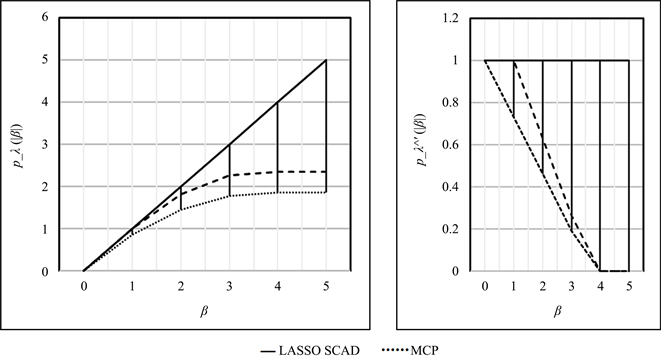Figure 1. LASSO, MCP and SCAD penalties when λ = 1 and a = 3.7

2.2. EM算法

${\mathcal{l}}^{c}\left(\phi ;{y}_{i},{z}_{i}\right)={\sum }_{i=1}^{n}\left\{{B}_{i}{W}_{i}{}^{\text{T}}\gamma -\mathrm{log}\left(1+{\text{e}}^{{W}_{i}{}^{\text{T}}\gamma }\right)+\left(1-{B}_{i}\right)\left[{y}_{i}{X}_{i}^{\text{T}}\beta -\left({y}_{i}+1\right)\mathrm{log}\left(1+{\text{e}}^{{X}_{i}^{\text{T}}\beta }\right)\right]\right\}$ (12)

$\begin{array}{l}p{\mathcal{l}}^{c}\left(\phi ;{y}_{i},{z}_{i}\right)=\underset{i=1}{\overset{n}{\sum }}\left\{{B}_{i}{W}_{i}^{\text{T}}\gamma -\mathrm{log}\left(1+\mathrm{exp}\left({W}_{i}{}^{\text{T}}\gamma \right)\right)\right\}-n\underset{k=1}{\overset{{p}_{1}}{\sum }}{p}_{{a}_{j}}|{\beta }_{j}|\\ \text{}+\underset{i=1}{\overset{n}{\sum }}\left(1-{B}_{i}\right)\left[{y}_{i}{X}_{i}^{\text{T}}\beta -\left({y}_{i}+1\right)\mathrm{log}\left(1+{\text{e}}^{{X}_{i}^{\text{T}}\beta }\right)\right]-n\underset{k=1}{\overset{{p}_{2}}{\sum }}{p}_{{b}_{k}}|{\gamma }_{k}|\end{array}$ (13)

1) E步：给定观测数据并假设当前的估计 ${\phi }^{\left(m\right)}$ 提供模型的真实参数，迭代m次时 $\delta$ 的条件期望由下式给出：

${\stackrel{^}{B}}_{i}{}^{\left(m\right)}=\left\{\begin{array}{l}{\left(\left(1+\mathrm{exp}{\left({W}_{i}^{\text{T}}{\stackrel{^}{\gamma }}^{\left(m\right)}\right)}^{-1}\right){\left(1+\mathrm{exp}\left({X}_{i}^{\text{T}}{\stackrel{^}{\beta }}^{\left(m\right)}\right)\right)}^{-1}\right)}^{-1},\text{}{y}_{i}=0\hfill \\ 0,\text{}{y}_{i}>0\hfill \end{array}$ (14)

$\mathcal{Q}\left(\phi |{\stackrel{^}{\phi }}^{\left(m\right)}\right)=E\left(p{\mathcal{l}}^{c}\left(\phi ;{y}_{i},{z}_{i}\right)|{y}_{i},{\stackrel{^}{\phi }}^{\left(m\right)}\right)={\mathcal{Q}}_{1}\left(\gamma |{\stackrel{^}{\phi }}^{\left(m\right)}\right)+{\mathcal{Q}}_{2}\left(\beta |{\stackrel{^}{\phi }}^{\left(m\right)}\right)$ (15)

${\mathcal{Q}}_{1}\left(\gamma |{\stackrel{^}{\phi }}^{\left(m\right)}\right)={\sum }_{i=1}^{n}{\stackrel{^}{B}}_{i}{}^{\left(m\right)}{W}_{i}^{\text{T}}\gamma -\mathrm{log}\left(1+\mathrm{exp}\left({W}_{i}^{\text{T}}\gamma \right)\right)-n{\sum }_{k=1}^{{p}_{1}}{p}_{{a}_{j}}|{\beta }_{j}|$ (16)

${\mathcal{Q}}_{2}\left(\beta |{\stackrel{^}{\phi }}^{\left(m\right)}\right)={\sum }_{i=1}^{n}\left(1-{\stackrel{^}{B}}_{i}{}^{\left(m\right)}\right)\left[{y}_{i}{X}_{i}^{\text{T}}\beta -\left({y}_{i}+1\right)\mathrm{log}\left(1+{e}^{{X}_{i}^{\text{T}}\beta }\right)\right]-n{\sum }_{k=1}^{{p}_{2}}{p}_{{b}_{k}}|{\gamma }_{k}|$ (17)

2) M步(关于 $\gamma$ )：

${\stackrel{^}{\gamma }}^{\left(m+1\right)}=\mathrm{arg}\underset{\gamma }{\underset{︸}{\mathrm{max}}}\mathcal{Q}\left(\gamma |{\stackrel{^}{\gamma }}^{\left(m\right)}\right)$ (18)

3) M步(关于 β )

${\stackrel{^}{\beta }}^{\left(m+1\right)}=\mathrm{arg}\underset{\beta }{\underset{︸}{\mathrm{max}}}\mathcal{Q}\left(\beta |{\stackrel{^}{\beta }}^{\left(m\right)}\right)$ (19)

${\mathcal{Q}}_{1}\left(\gamma |{\theta }^{\left(k\right)}\right)$ 是一个加权惩罚逻辑对数似然函数， ${\mathcal{Q}}_{2}\left(\beta |{\theta }^{\left(k\right)}\right)$ 是一个惩罚逻辑回归对数似然函数，重复E步和M步直到收敛。

2.3. 调整参数的选择

$BIC=-2\mathcal{l}\left(\stackrel{^}{\phi };{a}_{j},{b}_{k}\right)+\mathrm{log}\left(n\right)df$ (20)

3. 仿真模拟

3.1. 模型和数据生成

$\left\{\begin{array}{c}logit\left({\theta }_{i}\right)={\beta }_{1}{x}_{i1}+{\beta }_{2}{x}_{i2}+\cdots +{\beta }_{20}{x}_{i20}\\ logit\left({p}_{i}\right)={\gamma }_{1}{w}_{i1}+{\gamma }_{2}{w}_{i2}+\cdots +{\gamma }_{20}{w}_{i20}\end{array}$

$\beta =\left(1.5,0,0,0,-0.22,0,0,0,-0.25,0,0.20,0,0.30,-0.32,0,0,0,0.20,0,0,0\right)$

$\gamma =\left(-1.05,0,0.45,0,-0.3,0,0,0,0,0,-0.33,0,-0.39,0,0,0.3,0,0,0,0.36,0\right)$

$\beta =\left(1.5,0,0,0,-0.22,0,0,0,-0.25,0,0.20,0,0.30,-0.32,0,0,0,0.20,0,0,0\right)$

$\gamma =\left(-1.05,0,0.45,0,-0.3,0,0,0,0,0,-0.33,0,-0.39,0,0,0.3,0,0,0,0.36,0\right)$

$\beta =\left(1.10,0,0,0,-0.36,0,0,0,0,0,0,0,0,-0.32,0,0,0,0,0,0,0\right)$

$\gamma =\left(0.3,-0.48,0,0,0,0.4,0,0,0,0,0.44,0,0.44,0,0,0,0,0,0,0,0\right)$

$\beta =\left(1.10,0,0,0,-0.36,0,0,0,0,0,0,0,0,-0.32,0,0,0,0,0,0,0\right)$

$\gamma =\left(0.3,-0.48,0,0,0,0.4,0,0,0,0,0.44,0,0.44,0,0,0,0,0,0,0,0\right)$

3.2. 仿真结果

1) 在零膨胀几何模型中，无论是零部分还是几何部分，LASSO在均方误差和特异性上都比SCAD和MCP表现更好，在敏感性上相反，ZIGe-MCP和ZIGe-SCAD方法之间没有明显的差别。BE方法的结果因显著性水平而异：在显著性水平较小时( $\alpha =0.01$ )，用较小的均方误差产生了更精确的估计，但可能选择较少的变量，导致不太精确的灵敏度；在显著性水平较大时( $\alpha =0.1573$ )，BE方法保留了更多的预测变量并且提高了灵敏度。就均方误差而言，BE (0.01)与ZIGe-MCP和ZIGe-SCAD相比具有竞争力。然而在所有的模拟情况中，BE (0.01)在零部分的敏感度最小，在小样本情况下尤其明显。

2) 随着样本量的增加，所有方法都有较好的性能(均方误差值降低)，零分量的灵敏度随之增加。表中的均方误差被定义为一个比率，而不是绝对值。当相关性增加时，我们在表1和附表1或者表2和附表2的结果中并未表明敏感性有明显变化。

3) 图2图3展示了预测的对数似然值。惩罚的零膨胀几何模型比BE方法的预测效果要稍好点。在模拟1中，样本量为100时，LASSO惩罚的表现力相对来说最好的，SCAD没有优势，BE方法随着显著性水平的增加，预测效果逐渐变差；而当样本量增加为300时，几种惩罚方法的效果都有所提高。在模拟4中， $\rho$ 值变为0.8，零膨胀率由原来的29%变为55%，模型具有较好的预测能力。Table 1. Results of simulation 1 (ρ = 0.4)Table 2. Results of simulation 4 (ρ = 0.8)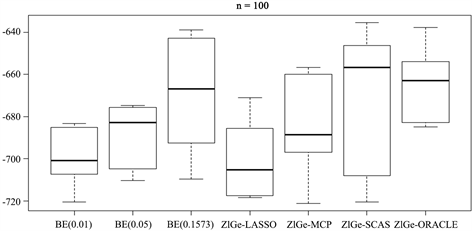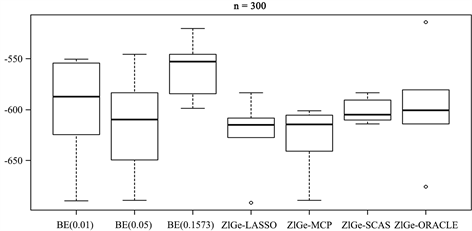Figure 2. The log-likelihood value of simulation 1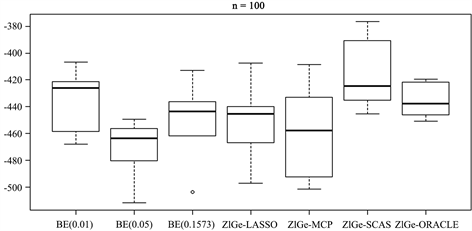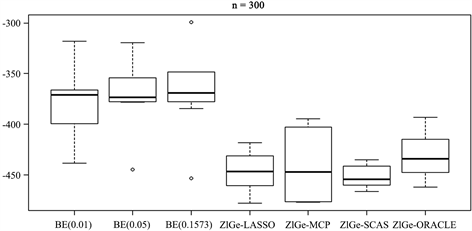Figure 3. The log-likelihood value of simulation 4

4. 讨论Table A1. Results of simulation 2 (ρ = 0.8)Table A2. Results of simulation 3 (ρ = 0.4)

  Cohen Jr., A.C. (1960) Estimating the Parameters of a Modified Poisson Distribution. Journal of the American Statistical Association, 55, 139-143. https://doi.org/10.1080/01621459.1960.10482054  Mullahy, J. (1986) Specification and Testing of Some Modified Count Data Models. Journal of Econometrics, 33, 341-365. https://doi.org/10.1016/0304-4076(86)90002-3  Lambert, D. (1992) Zero-Inflated Poisson Regression with an Application to Defects in Manufacturing. Technometrics, 34, 1-14. https://doi.org/10.2307/1269547  Lee, J.H., Han, G., Fulp, W.J., et al. (2012) Analysis of Overdispersed Count Data: Application to the Human Papillomavirus Infection in Men (HIM) Study. Epidemiology & Infection, 140, 1087-1094. https://doi.org/10.1017/S095026881100166X  Lee, S.M., Li, C.S., Hsieh, S.H., et al. (2012) Semiparametric Estimation of Logistic Regression Model with Missing Covariates and Outcome. Metrika, 75, 621-653. https://doi.org/10.1007/s00184-011-0345-9  Huang, L., Zheng, D., Zalkikar, J., et al. (2017) Zero-Inflated Poisson Model Based Likelihood Ratio Test for Drug Safety Signal Detection. Statistical Methods in Medical Research, 26, 471-488. https://doi.org/10.1177/0962280214549590  Liu, H. (2007) Growth Curve Models for Zero-Inflated Count Data: An Application to Smoking Behavior. Structural Equation Modeling: A Multidisciplinary Journal, 14, 247-279. https://doi.org/10.1080/10705510709336746  肖翔, 刘福窑. 零膨胀几何分布的参数估计[J]. 上海工程技术大学学报, 2018, 32(3): 267-271+277.  肖翔. 0-1膨胀几何分布回归模型及其应用[J]. 系统科学与数学, 2019, 39(9): 1486-1499.  Breiman, L. (1995) Better Subset Regression Using the Nonnegative Garrote. Technometrics, 37, 373-384. https://doi.org/10.1080/00401706.1995.10484371  Shen, X. and Ye, J. (2002) Adaptive Model Selection. Journal of the American Statistical Association, 97, 210-221. https://doi.org/10.1198/016214502753479356  Hoerl, A.E. and Kennard, R.W. (1970) Ridge Regression: Biased Estimation for Nonorthogonal Problems. Technometrics, 12, 55-67. https://doi.org/10.1080/00401706.1970.10488634  Frank, L.L.E. and Friedman, J.H. (1993) A Statistical View of Some Chemometrics Regression Tools. Technometrics, 35, 109-135. https://doi.org/10.1080/00401706.1993.10485033  Tibshirani, R. (1996) Regression Shrinkage and Selection via the Lasso. Journal of the Royal Statistical Society: Series B (Methodological), 58, 267-288. https://doi.org/10.1111/j.2517-6161.1996.tb02080.x  Meinshausen, N. (2007) A Note on the Lasso for Gaussian Graphical Model Selection. Statistics and Probability Letters, 78, 880-884. https://doi.org/10.1016/j.spl.2007.09.014  Fan, J. and Li, R. (2001) Variable Selection via Nonconcave Penalized Likelihood and Its Oracle Properties. Journal of the American Statistical Association, 96, 1348-1360. https://doi.org/10.1198/016214501753382273  Fan, J. and Li, R. (2006) Statistical Challenges with High Dimensionality: Feature Selection in Knowledge Discovery. Proceedings of the International Congress of Mathematicians, Madrid, 22-30 August 2006, 595-622.  Zou, H. and Hastie, T. (2005) Regularization and Variable Selection via the Elastic Net. Journal of the Royal Statistical Society: Series B (Statistical Methodology), 67, 301-320. https://doi.org/10.1111/j.1467-9868.2005.00503.x  Zhang, C.H. (2010) Nearly Unbiased Variable Selection under Minimax Concave Penalty. The Annals of Statistics, 38, 894-942. https://doi.org/10.1214/09-AOS729  Buu, A., Johnson, N.J., Li, R., et al. (2011) New Variable Selection Methods for Zero-Inflated Count Data with Applications to the Substance Abuse Field. Statistics in Medicine, 30, 2326-2340. https://doi.org/10.1002/sim.4268  Wang, Z., Ma, S., Wang, C.Y., et al. (2014) EM for Regularized Zero-Inflated Regression Models with Applications to Postoperative Morbidity after Cardiac Surgery in Children. Statistics in Medicine, 33, 5192-5208. https://doi.org/10.1002/sim.6314  Chen, T., Wu, P., Tang, W., et al. (2016) Variable Selection for Distribution-Free Models for Longitudinal Zero-Inflated Count Responses. Statistics in Medicine, 35, 2770-2785. https://doi.org/10.1002/sim.6892